# Precalculus : Translations

## Example Questions

### Example Question #11 : Graphing Functions

If the graph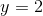was translated two units to the right and two units down, what is the new equation?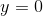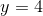Explanation:

Do not confuse the movement of an equation of a line with the movement of a point.  Movingtwo units to the right has no effect on the equation of the horizontal line.  Moving the equation two units down will change the horizontal line equation to.

### Example Question #1 : Translations

Which of the following expressions corresponds to a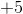vertical translation of f(x)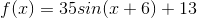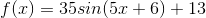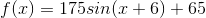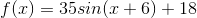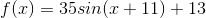Explanation:

Which of the following expressions corresponds to avertical translation of f(x)In my experience, vertical translations are the easiest way of changing a graph. A vertical translation will be of the form: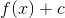What this basically means is that we simply add our vertical translation onto the end of our function. This will simply make our function +c higher. In this case we need a vertical translation of positive 5. Do the following: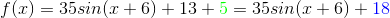### Example Question #1 : Transformations

Which of the following will shift any general function f(x) down 7 and left 16?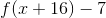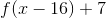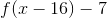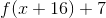Explanation:

Which of the following will shift any general function f(x) down 7 and left 16?

Recall that to shift any function vertically, we simply add or subtract a number on the end of the function. Because we are going down 7, we want to subtract 7 from the end. So far we have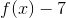Next, to translate horizontally, we need to add or subtract a number within f(x) itself. This means that wherever we have an x, we need to replace it with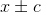.

Further, we need to realize that horizontal translations work backwards from what you might expect. If we do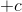, we will shift to the left. If we do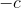, we will shift to the right.

So for this problem, we need to do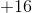, giving us:### Example Question #2 : Translations

Which of the following represents a horizontal shift of g(x) 3 to the right?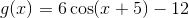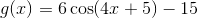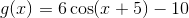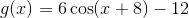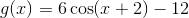Explanation:

Which of the following represents a horizontal shift of g(x) 3 to the right?Horizontal translations take the form of: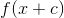The key thing to remember however, is that horizontal translations are a little counterintuitive. A positive "c" will move the function left, whereas a negative "c" will move the function to the right. (Maybe not what you'd expect)

So, if we are going to shift our function 3 to the right, we will do the following: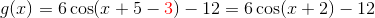So we get:### Example Question #2 : Translations

Choose the function whose vertex is on the origin.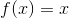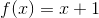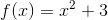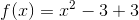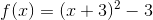Explanation:

Each graph you have ever seen is derived from a parent function which is the most basic form of that type of graph. For example,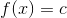is a straight line across the real number c,is a straight diagonal line from bottom left to top right through the origin, and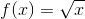is a curved line starting from the origin and curving up and to the right (note this list is not all inclusive). Use a graphing utility to verify a few of these so you can see. When a function is not in its most basic parent form it has been shifted, reflected, stretched or compressed based on various changes to the parent function.

The basic rules for horizontal and vertical shifts are as follows:

if c is a positive real number, vertical and horizontal shifts in a function f(x) can be accompolished by:

1) vertical shift c units up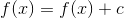2) c units down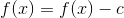3) horizontal shift c units to the right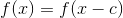4) c units to the left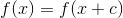*note the opposite shift in the horizontal shifts does not follow the traditional number line.will be shifted 3 units to the left (rule number 4) and then 3 units down (rule number 3) so its vertex will not be at the origin.will only be shifted up 3 units (rule number 1) from the origin.does not have a vertex since it is a straight line.is also a straight line, and it will not pass through the origin since it is shifted up 1 unit (rule number 1) .has a net movement of 0 units since the two shifts of 3 down and then 3 up cancel each other out (rule number 1) so its vertex will remain on at the origin and is therefore the correct answer.

### All Precalculus Resources